Courses

# Mathematics Test 3 - Entire XI Syllabus

## 25 Questions MCQ Test Mock Test Series for JEE Main & Advanced 2022 | Mathematics Test 3 - Entire XI Syllabus

Description
This mock test of Mathematics Test 3 - Entire XI Syllabus for JEE helps you for every JEE entrance exam. This contains 25 Multiple Choice Questions for JEE Mathematics Test 3 - Entire XI Syllabus (mcq) to study with solutions a complete question bank. The solved questions answers in this Mathematics Test 3 - Entire XI Syllabus quiz give you a good mix of easy questions and tough questions. JEE students definitely take this Mathematics Test 3 - Entire XI Syllabus exercise for a better result in the exam. You can find other Mathematics Test 3 - Entire XI Syllabus extra questions, long questions & short questions for JEE on EduRev as well by searching above.
QUESTION: 1

### Value of limx → 0⁡(1+Sin(x))Cosec(x)

Solution:

limx → 0⁡(1+Sin(x))Cosec(x)

Put sin(x) = t we get

limt → 0⁡(1+t)(1t) = e.

QUESTION: 2

### The number of straight lines that can be drawn out of 10 points of which 7 are collinear is

Solution:
1. One line passing through 7 collinear points
2. 7 lines passing from each collinear point to non collinear one (3 times as there are 3 non-collinear points)
3. 3 lines joining each pair of non-collinear points 1+(7*3)+3 1+21+3 =25
QUESTION: 3

### If the correlation coefficient between two variables is 1, then the two least square lines of regression are

Solution:
QUESTION: 4

The real part of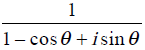is

Solution:

First we consider theta as x
1/1-cosx+isinx=1/2cos^2 x/2 + 2isinx/2cosx/2
=sec(x/2)/2 {1/cosx/2 + isinx/2}
=sec(x/2)/2 (1/e^ix/2). (e^ix=cosx+isinx){eulers theorem)
=sec(x/2)/2 e^-ix/2
=sec(x/2)/2 [ cos(-x/2) + i sun(-x/2)]
=1/2secx/2.cosx/2.-1/2itanx/2
=1/2.-i(1/2tanx/2)
=Re(z)=1/2

QUESTION: 5

The value of   log3 tan1º + log3 tan2º +....+log3 tan 89º   is

Solution:

Loga+log=log(ab)
tan(90-A)=cotA
log(tab.tan2......tan45........cot2.cot1). tan45=1
tanA.cotA=1
=log(1)=0

QUESTION: 6

If the solution of quadratic equation x2-11x+22 are x=3 and x=6, then the base of the number is

Solution:
QUESTION: 7

12 + (12 + 22) + (12 + 22 + 32 )+..... upto 22nd term is

Solution:
QUESTION: 8

In ΔABC, ∠B = 90o and b a = 4. The area of the triangle is the maximum when ∠C  is

Solution:
QUESTION: 9

Let E be the ellipse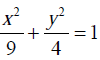and C be the circle x2 y2  = 4. Let P and Q be the point (1,2) and (2,1) respectively. Then

Solution:
QUESTION: 10

The third term of a G.P. is 4. The products of the first five term is

Solution:
QUESTION: 11
The sum of the infinIte of terms of G.P. is 20, and the sum of their square is 100, then the first term of
Solution:
QUESTION: 12

If cos-1 x/2 + cos -1 y/3 = θ Then 9x2 - 12xy cos θ + 4y2  is

Solution:
QUESTION: 13

The foci of the ellipse 25 (x+1)2 + 9(y+2)2 = 225 are at

Solution:
QUESTION: 14

If  A1, A2  be two A.M’s and G1, G2 be two G..M.’s between a and b, then ( A1, A)/G1,G2 is equal to

Solution:
QUESTION: 15

A tree is broken by wind, its upper part touches the ground at a point 10 meters from the foot of the tree and makes an angle of 60º with the ground the entire lenght of the tree of

Solution:
QUESTION: 16
How many diagonals can be drawn in a polygon of 15 sides ?
Solution:
QUESTION: 17

9x2 - 16y2 = 144  represents

Solution:
QUESTION: 18

If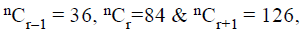then r is equal to

Solution:
QUESTION: 19

Coefficient of X4 in the expansion of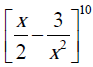is

Solution:
QUESTION: 20

The locus of the mid-point of the chords of a circle x2 + y2 =4, which subtended a right angle at the centre is

Solution:
*Answer can only contain numeric values
QUESTION: 21

If α and β are roots of eq. λ(x2–x) + x + 5 = 0
If λ1, λ2 are two values of λ for which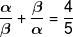then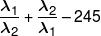Solution: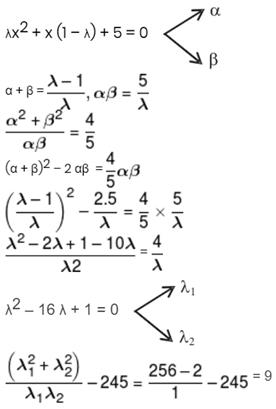*Answer can only contain numeric values
QUESTION: 22

If graph of x2 + bx + d is following.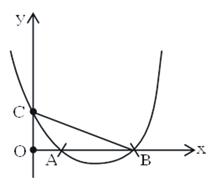ΔOBC is right angled isosceles triangle and A is mid point of OB then d =

Solution:

f(0) = d
OC = d ⇒    OB = d
OA = d/2
Roots of eq. are d & d/2
Product of roots d × d/2 = d

*Answer can only contain numeric values
QUESTION: 23

Sum of non real roots of eq.
(x2 + x – 2) · (x2 + x – 3) = 12 is …..

Solution:

x2 + x = t
t2 – 5t – 6 = 0
t = 6 or t = –1
x2 + x = 6             x2 + x = –1
x2 + x – 6 = 0       x2 + x + 1 = 0
Roots are real      sum of roots = –1

*Answer can only contain numeric values
QUESTION: 24

21/4 . 41/8 . 81/16-------- ∞ is equal to?

Solution: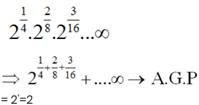*Answer can only contain numeric values
QUESTION: 25

If ƒ(x) = |x – 3| + |x – 16| + |22 – x|, ∀ x ∈ R increases in (a,∞), then a/4 is equal to

Solution:

⇒ ƒ'(x) > 0 ∀ x ≥ 16  ⇒ a = 16